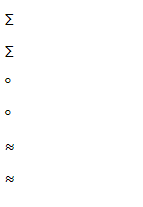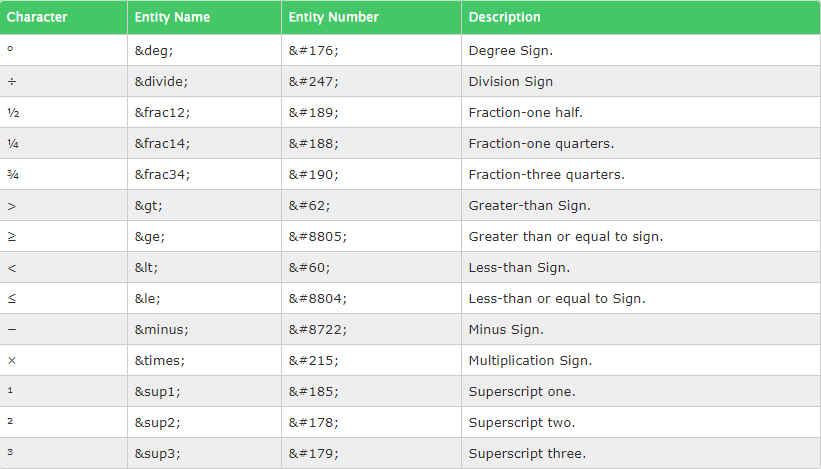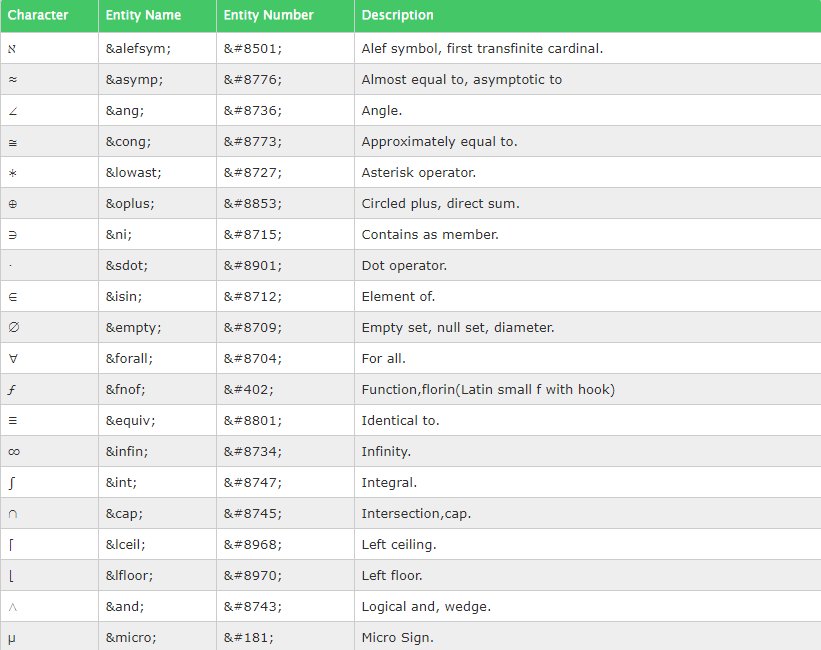Related Articles
HTML5 | Mathematical operators
• Difficulty Level : Basic
• Last Updated : 03 Dec, 2018

Mathematical Operators are used for representation of mathematical and technical operators. In HTML5 some special codes are used. For the representation of these operators we can use the name or hexadecimal(Hex) reference.

Examples:

```Input : &minus or &#8722
Output : -
Input : &times or &#215
Output : x
Input : &frac34 or &#190
Output : 3/4
```
 ```````  ` `  ``

``  ``

&#``8721``

``  ``

°

``  ``

&#``176``

``  ``

``  ``

&#``8776``

`````

Output:

``````

In writing the hex reference in html code, a semicolon is required at the end.
Some Common mathematical Characters are-Some Complex mathematical symbols are-My Personal Notes arrow_drop_up